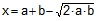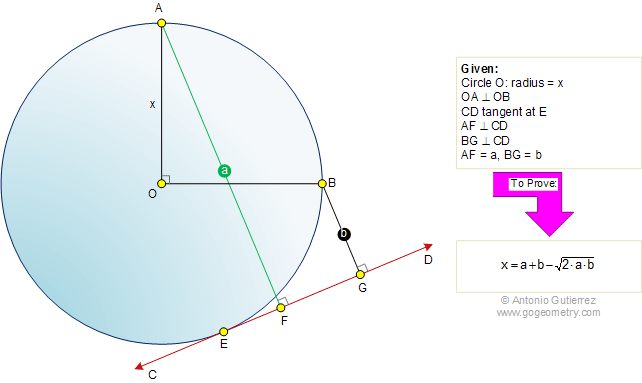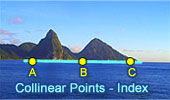# Geometry Problem 460: Circle, Tangent, Perpendicular, Radius, Distance, Measurement

 The figure shows a circle O with the radius OA perpendicular to radius OB. Line CD is tangent to circle O at E. AF and BG are perpendicular to CD. If OA = x, AF = a, and BG = b, prove that.More Problems: Proposed Problem 461. Three Circles, Tangent, Right Angle, Center, Distance, Measurement. Proposed Problem 459. Right triangle, Squares, Distance, Measurement. Proposed Problem 458. Square, Semicircle, Circular Sector, Internal Common Tangent, Measurement. Proposed Problem 457. Triangle, First Brocard Point, Congruent angles, Circle, Circumradius.Home | Search | Geometry | Problems | All Problems | Visual Index | 451-460 | Email | Post a comment or solution | By Antonio Gutierrez